# Standard measurements

In the cursor window of each graph and in meters, various different measurements can be selected. These measurements include:

Icon Measurement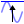Maximum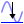Minimum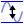Maximum-Minimum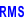RMS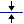Mean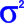Variance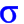Standard Deviation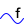Frequency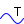Period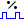Duty cycle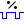Duty cycle inverted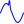Crest factor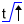Rise time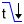Fall time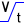Slewrate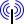dBm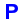Power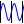Period count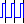Pulse count (positive)Pulse count (negative)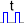Pulse width (positive)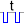Pulse width (negative)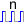Rising edge count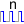Falling edge count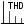Total Harmonic Distortion (THD)

In the cursor window the following additional measurements are possible:

Icon Measurement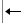Left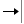Right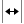Right-Left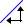Slope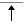Top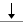Bottom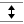Top-Bottom

## Short description

This section gives a short description of the standard measurements for the meter and cursors.

The measurements are calculated over a sample range. When using the cursor window with the vertical cursors switched on, the sample range is equal to the samples in between the left and right cursor. When the vertical cursors are switched off, or in a meter object, the sample range is equal to the post samples.

Some measurements are explained with a formula. In these formulas the following applies:

• m corresponds to the index of the first sample in the sample range
• n corresponds to the index of the last sample in the sample range
• N is the number of samples in the sample range and is equal to n - m + 1
• xi corresponds to the ith sample

### Maximum

The measurementMaximum is the highest value in the sample range.

### Minimum

The measurementMinimum is the lowest value in the sample range.

### Maximum-Minimum

The measurementMaximum-Minimum, also known as peak-peak is the highest value in the sample range minus the lowest value.

### Root Mean Square (RMS)

The measurementRMS is equal to the square root of the mean of the squares of all samples in the sample range: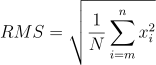### Mean

The measurementMean is the mean value of all samples in the sample range.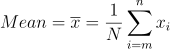### Variance

The measurementVariance is a measure of how values are distributed around the mean value.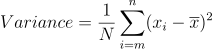### Standard Deviation

The measurementStandard deviation (σ) is equal to the square root of the variance. The standard deviation is equal to the RMS value for signals with a zero mean value (AC signals).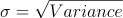### Frequency

The measurementFrequency determines the frequency of a time based signal. The frequency is determined by searching the rising slopes in a signal and measuring the time between them.

For a correct measurement, at least two rising slopes must be present in the sample range.

### Period

The measurementPeriod determines the period time of a time based signal. The period time is determined by searching the rising slopes in a signal and measuring the time between them.

For a correct measurement, at least two rising slopes must be present in the sample range.

### Duty cycle

The measurementDuty cycle is defined as the ratio between the time that a signal is higher than half the amplitude and the period. It is expressed as a percentage.

### Duty cycle inverted

The measurementDuty cycle inverted is defined as the ratio between the time that a signal is lower than half the amplitude and the period. It is expressed as a percentage.

### Crest factor

TheCrest factor is equal to the peak amplitude of a waveform divided by the RMS value.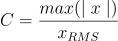The Crest factor can be used to get an idea of the quality of a signal. A signal with more peaks will have a higher Crest factor. The following table lists some Crest factors for some ideal standard signals.

Signal typeCrest factor
Sine √2 ≈ 1.414
Triangle √3 ≈ 1.732
Block 1
DC 1

### Rise time

TheRise time is the time it takes for the signal to rise from 10% to 90% of its top-bottom value. The first rising slope in the sample range is used.

### Fall time

TheFall time is the time it takes for the signal to fall from 90% to 10% of its top-bottom value. The first falling slope in the sample range is used.

### Slew rate

TheSlew rate is defined as the change of voltage per unit of time and is expressed in V/s. The first edge in the sample range is used.

### dBmdBm is the power ratio in decibels of the measured power referenced to one milliwatt. The following formula is used, in which an adjustable virtual reference resistor Rref is used to convert the measured voltage to power. The default value of Rref is 600Ω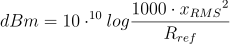### PowerPower shows the amount of electrical power that is dissipated in a virtual reference resistor by the measured signal. The following formula is used, in which an adjustable virtual reference resistor Rref is used to convert the measured voltage to power. The default value of Rref is 600Ω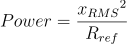### Period countPeriod count determines the number of periods in a signal. It uses the mid level crossings in the signal to determine the amount of periods.

For a correct measurement, at least three mid level crossings must be present in the sample range.

### Pulse count (positive)Pulse count (positive) determines the number of rising pulses in a signal. It uses the mid level crossings in the signal to determine the amount of pulses.

For a correct measurement, at least two mid level crossings must be present in the sample range.

### Pulse count (negative)Pulse count (negative) determines the number of falling pulses in a signal. It uses the mid level crossings in the signal to determine the amount of pulses.

For a correct measurement, at least two mid level crossings must be present in the sample range.

### Pulse width (positive)Pulse width (positive) determines the width of rising pulses in a signal. It uses the mid level crossings in the signal to determine the width of pulses.

For a correct measurement, at least two mid level crossings must be present in the sample range. When multiple pulses occur in the sample range, the average width of all detected pulses is shown.

### Pulse width (negative)Pulse width (negative) determines the width of falling pulses in a signal. It uses the mid level crossings in the signal to determine the width of pulses.

For a correct measurement, at least two mid level crossings must be present in the sample range. When multiple pulses occur in the sample range, the average width of all detected pulses is shown.

### Rising edge countRising edge count determines the number of rising edges in a signal. It uses the mid level crossings in the signal to determine the amount of edges.

For a correct measurement, at least two mid level crossings must be present in the sample range.

### Falling edge countFalling edge count determines the number of falling edges in a signal. It uses the mid level crossings in the signal to determine the amount of edges.

For a correct measurement, at least two mid level crossings must be present in the sample range.

### Total Harmonic Distortion (THD)

The Total Harmonic Distortion (THD) is defined as the ratio between the power of the harmonic frequencies above the base frequency and the power of the base frequency. This ratio is displayed in dB. It is a measure of the distortion in a signal.

The THD is calculated using the following formula: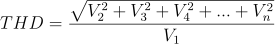where V1 is the RMS amplitude of the base frequency and V2 to Vn are the RMS amplitudes of each higher harmonic.

The THD measurement can only be used on frequency based signals or spectra.

### Left

The measurementLeft can be used in the cursor window. It is the value of the signal at the position of the left cursor.

### Right

The measurementRight can be used in the cursor window. It is the value of the signal at the position of the right side cursor.

### Right-Left

The measurementRight-Left can be used in the cursor window. It is the difference between the magnitude of the signal at the position of the right and left cursor.

### Slope

TheSlope of a signal is the change of magnitude divided by the elapsed time. The measurement slope determines the slope in a signal between the left and the right cursor.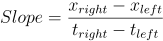### Top

The measurementTop can be used in the cursor window. It represents the value of the signal at the position of the top cursor.

### Bottom

The measurementBottom can be used in the cursor window. It represents the value of the signal at the position of the bottom cursor.

### Top-Bottom

The measurementTop-Bottom can be used in the cursor window. It represents the difference between the signal magnitudes at the positions of the top and bottom cursor.

## Reference resistor

A user configurable virtual impedance is available that is used by the Power measurementand the dBm measurement. Cicking the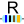Impedance button in the cursor readout window will allow to set its value. Its default value is 600 Ω.